# CBSE Solutions for Class 10 Maths

#### Select CBSE Solutions for class 10 Subject & Chapters Wise :

The angle of elevation from ground to the top of the building is 30° and the distance between the point and the base of the building is 10m. Find the height of the building.

tanx = Height/Base

tan30° = Height/10

Height= 10/√3m

The angle of elevation from ground to the top of the building is 30° and the height of the building is 10/3m. Find the distance between the point and the base of the building.

tanx = Height/Base

tan30° =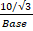Base = 10

The angle of elevation from ground to the top of the building is 60° and the distance between the point and the base of the building is 10m. Find the height of the building.

tanx = Height/Base

Tan60° = Height/10

Height= 103m

If the distance between the base of the building and the point is 10m and the height of the building is 10/3m. Find the angle of elevation from ground to the top of the building.

tanx = Height/Base

tanx=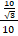= 1/3

x= 30°

The angle of elevation from ground to the top of the building is 60° and the height of the building is 103m. Find the distance between the point and the base of the building.

tanx = Height/Base

tan60° =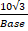Base = 10

If the distance between the base of the building and the point is 10m and the height of the building is 103m. Find the angle of elevation from ground to the top of the building.

tanx = Height/Base

tanx=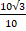= 3

x= 60°

The angle of elevation from ground to the top of the building is 60° and the height of the building is 5√3m. Find the distance between the point and the base of the building.

tanx = Height/Base

tan60° =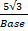Base = 5

The angle of elevation from ground to the top of the building is 30° and the distance between the point and the base of the building is 5m. Find the height of the building.

tanx = Height/Base

tan30° = Height/5

Height= 5/3m

The angle of elevation from ground to the top of the building is 60° and the distance between the point and the base of the building is 5m. Find the height of the building.

tanx = Height/Base

Tan60° = Height/5

Height= 5√3m

The angle of elevation from ground to the top of the building is 30° and the height of the building is 5/√3m. Find the distance between the point and the base of the building.

tanx = Height/Base

tan30° =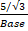Base = 5

A ladder 15 m long just reaches the top of a vertical wall. If the ladder makes an angle of 60° with the wall, then calculate the height of the wall. (2013OD)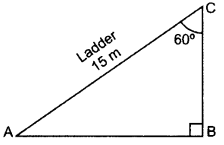BAC = 180° – 90° – 60° = 30°
sin 30° = BC/AC
1/2=BC/15
2BC = 15
BC = 15/2m

In the given figure, a tower AB is 20 m high and BC, its shadow on the ground, is 20√3m long. Find the Sun’s altitude. (2015OD)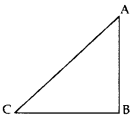AB = 20 m, BC =20√3m,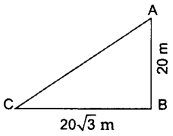In ∆ABC, Using trigonometry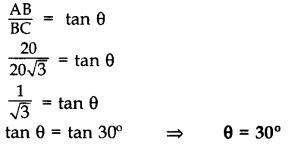​​​​​​​

In the figure, AB is a 6 m high pole and CD is a ladder inclined at an angle of 60° to the horizontal and reaches up to a point D of pole. If AD = 2.54 m. Find the length of the ladder. (Use √3 = 1.73) (2016D)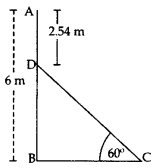BD = AB – AD = 6 – 2.54 = 3.46 m
In rt., ∆DBC, sin 60° = BD/DC
√3/2=3.46/DC
√3 /DC = 3.46 x 2
Length of the ladder, DC = 6.92 /√3=6.92/1.73
DC = 4 m

A ladder, leaning against a wall, makes anangle of 60° with the horizontal. If the foot of the ladder is 2.5 m away from the wall, find the length of the ladder. (2016OD)

Let us assume AC be the ladder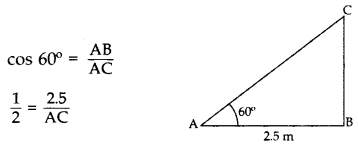Length of ladder, AC = 5 m 2.5 m

If a tower 30 m high, casts a shadow 10√3m long on the ground, then what is the angle of elevation of the sun? (2017OD)

Let us assume required angle be θ.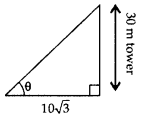tan θ = 30 /10 √3
tan θ = √3
tan θ = tan 60°

θ = 60°A=30° and AB=3cm

 1    ∠C A    2√3  cm 2    AC B    √3 cm 3    BC C    60° 4    ∠B D    90°

1-C, 2-A, 3-B, 4-DC=60° and BC= 3 cm

 1 ∠A A 2√3  cm 2 AB B 30° 3 AC C 90° 4 ∠B D 3cm

1-B, 2-D, 3-A, 4-CC=45° and BC= 3 cm

 1 ∠A A 90° 2 AB B 45° 3 AC C 3 cm 4 ∠B D √6

1-B, 2-C, 3-D, 4-A